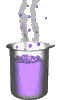Science class online
Chemistry and physics lessons

# Mechanics

Free online mechanics lesson for elementary school,  middle school and high school.Science class Chemistry Electricity Optics Mechanics Mechanics lessons Gravity - The solar system - motions of celestial bodies - Physical interactions - Effects of gravity - Weight of an object - Difference between weight and mass - Relationship between weight and mass  Energy - Energy basics - Potential energy - Kinetic energy - Mechanical energy - Translational Kinetic Energy - kinetic energy mass and speed

 _____________________________________________________   _____________________________________________________

## Gravity

### Kinetic energy

 1) What is kinetic energy ? An object owns kinetic energy when it's moving: kinetic energy is the motion energy. Its symbol is KE and its unit is joule (J). This energy depends on speed: the faster an object moves, the more it has kinetic energy A stationary object therefore does not have kinetic energy. ______________________________________ ______________________________________ 2) Kinetic energy of a falling object A falling ball is going faster and faster until it reaches the ground. As speed increases, kinetic energy increases too so where does this energy come from ? While the ball is dropping, the height decreases, therefore potential energy decreases too: Potential energy is converted into kinetic energy. When the ball reaches the ground, all its initial potential energy has been converted in kinetic energy and then this kinetic energy is transfered to the ground. ______________________________________ ______________________________________
____________________________________

____________________________________